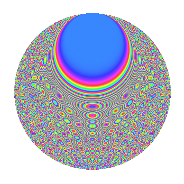# Properties

 Label 1890.2.btLevel 1890 Weight 2 Character orbit bt Rep. character $$\chi_{1890}(331,\cdot)$$ Character field $$\Q(\zeta_{9})$$ Dimension 576 Sturm bound 864

# Learn more about

## Defining parameters

 Level: $$N$$ = $$1890 = 2 \cdot 3^{3} \cdot 5 \cdot 7$$ Weight: $$k$$ = $$2$$ Character orbit: $$[\chi]$$ = 1890.bt (of order $$9$$ and degree $$6$$) Character conductor: $$\operatorname{cond}(\chi)$$ = $$189$$ Character field: $$\Q(\zeta_{9})$$ Sturm bound: $$864$$

## Dimensions

The following table gives the dimensions of various subspaces of $$M_{2}(1890, [\chi])$$.

Total New Old
Modular forms 2640 576 2064
Cusp forms 2544 576 1968
Eisenstein series 96 0 96

## Trace form

 $$576q$$ $$\mathstrut -\mathstrut 6q^{6}$$ $$\mathstrut -\mathstrut 24q^{9}$$ $$\mathstrut +\mathstrut O(q^{10})$$ $$576q$$ $$\mathstrut -\mathstrut 6q^{6}$$ $$\mathstrut -\mathstrut 24q^{9}$$ $$\mathstrut -\mathstrut 24q^{11}$$ $$\mathstrut +\mathstrut 6q^{14}$$ $$\mathstrut +\mathstrut 48q^{17}$$ $$\mathstrut +\mathstrut 24q^{21}$$ $$\mathstrut -\mathstrut 36q^{23}$$ $$\mathstrut -\mathstrut 6q^{29}$$ $$\mathstrut +\mathstrut 72q^{33}$$ $$\mathstrut -\mathstrut 6q^{36}$$ $$\mathstrut +\mathstrut 12q^{39}$$ $$\mathstrut +\mathstrut 12q^{41}$$ $$\mathstrut -\mathstrut 6q^{45}$$ $$\mathstrut -\mathstrut 36q^{47}$$ $$\mathstrut -\mathstrut 36q^{49}$$ $$\mathstrut +\mathstrut 72q^{51}$$ $$\mathstrut +\mathstrut 72q^{54}$$ $$\mathstrut +\mathstrut 6q^{56}$$ $$\mathstrut +\mathstrut 48q^{57}$$ $$\mathstrut +\mathstrut 120q^{59}$$ $$\mathstrut -\mathstrut 18q^{61}$$ $$\mathstrut +\mathstrut 96q^{62}$$ $$\mathstrut +\mathstrut 36q^{63}$$ $$\mathstrut -\mathstrut 288q^{64}$$ $$\mathstrut +\mathstrut 12q^{65}$$ $$\mathstrut +\mathstrut 108q^{69}$$ $$\mathstrut +\mathstrut 18q^{70}$$ $$\mathstrut -\mathstrut 48q^{71}$$ $$\mathstrut -\mathstrut 144q^{73}$$ $$\mathstrut +\mathstrut 72q^{74}$$ $$\mathstrut +\mathstrut 60q^{77}$$ $$\mathstrut -\mathstrut 96q^{78}$$ $$\mathstrut +\mathstrut 36q^{79}$$ $$\mathstrut +\mathstrut 12q^{80}$$ $$\mathstrut -\mathstrut 24q^{81}$$ $$\mathstrut +\mathstrut 6q^{84}$$ $$\mathstrut -\mathstrut 72q^{85}$$ $$\mathstrut +\mathstrut 48q^{86}$$ $$\mathstrut +\mathstrut 120q^{87}$$ $$\mathstrut -\mathstrut 72q^{91}$$ $$\mathstrut -\mathstrut 36q^{92}$$ $$\mathstrut +\mathstrut 48q^{93}$$ $$\mathstrut +\mathstrut 36q^{94}$$ $$\mathstrut +\mathstrut 48q^{98}$$ $$\mathstrut +\mathstrut O(q^{100})$$

## Decomposition of $$S_{2}^{\mathrm{new}}(1890, [\chi])$$ into irreducible Hecke orbits

The newforms in this space have not yet been added to the LMFDB.

## Decomposition of $$S_{2}^{\mathrm{old}}(1890, [\chi])$$ into lower level spaces

$$S_{2}^{\mathrm{old}}(1890, [\chi]) \cong$$ $$S_{2}^{\mathrm{new}}(189, [\chi])$$$$^{\oplus 4}$$$$\oplus$$$$S_{2}^{\mathrm{new}}(378, [\chi])$$$$^{\oplus 2}$$$$\oplus$$$$S_{2}^{\mathrm{new}}(945, [\chi])$$$$^{\oplus 2}$$